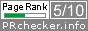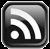# LEARN MS-ACCESS TIPS AND TRICKS

Learn Microsoft Access Advanced Programming Techniques, Tips and Tricks.

### Introduction.

How to find number of work-days (excluding Saturdays and Sundays) from a date-range in Microsoft Access?

The logic is simple, first find out how many whole weeks are there in the date range. Multiplying whole-weeks by 5 gives the number of work-days from whole weeks.  From the remaining days find and exclude Saturdays and Sundays, if any. Add the remaining days to the total work-days.

DateDiff(), DateAdd() functions are used for calculations and the Format() function gets day-of-the-week in three character form to find Saturday and Sunday to exclude from the remaining days.

Find the VBA Code segments for the above steps below, and full VBA Work_Days() Function Code at the end of this Article.

1. Find number of Whole Weeks between Begin-Date and End-Date:
`WholeWeeks = DateDiff("w", BeginDate, EndDate)`

WholeWeeks * 5 (7 - Saturdays & Sundays) will give the number of working days in whole weeks. Now, all that remains to find is how many working days left in the remaining days, if any?

2. Find the date after the whole week days:
`DateCnt = DateAdd("ww", WholeWeeks, BeginDate)`
3. Find number of work days in the remaining days by checking and excluding Saturdays and Sundays:
```Do While DateCnt <= EndDate
If Format(DateCnt, "ddd") <> "Sun" And _
Format(DateCnt, "ddd") <> "Sat" Then
EndDays = EndDays + 1
End If
DateCnt = DateAdd("d", 1, DateCnt)'increment the date by 1
Loop
```
4. Calculate the Total Workdays:
`Work_Days = Wholeweeks * 5 + EndDays`

### The Whole Calculation in Work_Days Function.

The full VBA Code of the Work_Days() Function is given below:

```Function Work_Days(BegDate As Variant, EndDate As Variant) As Integer

Dim WholeWeeks As Variant
Dim DateCnt As Variant
Dim EndDays As Integer

On Error GoTo Err_Work_Days

BegDate = DateValue(BegDate)
EndDate = DateValue(EndDate)
'Number of whole weeks
WholeWeeks = DateDiff("w", BegDate, EndDate)
'Next date after whole weeks of 7 days each
EndDays = 0 'to count number of days except Saturday & Sunday

Do While DateCnt <= EndDate
If Format(DateCnt, "ddd") <> "Sun" And _
Format(DateCnt, "ddd") <> "Sat" Then
EndDays = EndDays + 1
End If
DateCnt = DateAdd("d", 1, DateCnt)'increment the date by 1
Loop
'Calculate total work days and return the result
Work_Days = WholeWeeks * 5 + EndDays

Exit Function

Err_Work_Days:

' If either BegDate or EndDate is Null, return a zero
' to indicate that no workdays passed between the two dates.

If Err.Number = 94 Then
Work_Days = 0
Exit Function
Else
' If some other error occurs, provide a message.
MsgBox "Error " & Err.Number & ": " & Err.Description
End If

End Function
```

The above VBA Code was taken from Microsoft Access Help Document.

Share:

Comments subject to moderation before publishing.

## TranslateSubscribe in a readerYour email address:

Delivered by FeedBurner